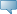## Catalog Entries

Current
Feb 22, 2020Select the Course Number to get further detail on the course. Select the desired Schedule Type to find available classes for the course.

 MAT 380 - Geometry Geometry Prerequisite: Math 230 This course offers a rigorous development of the core concepts and principles of geometry with an emphasis on proof and extensive problem solving. Topics include: axioms of Euclidean geometry, basic figures in two- and three- dimensions and their classification, congruence criteria for triangles, relationships between sides and angles in a triangle, parallel lines, the parallel postulate and its implications, basic properties of non-Euclidean geometry in two dimentsions (hyperbolic and spherical), basic constructions in the plane, similarity, right triangles and trigonometry, the Pythagorean theorem and other algebraic relationships in triangles, plane transformations and symmetry, area and volume of two and three dimensional figures. Geometry software is used as a technology tool for visualization and exploration in the plane. 3.000 Credit hours 3.000 Lecture hours Levels: Undergraduate Schedule Types: Lecture Mathematical Sciences Department